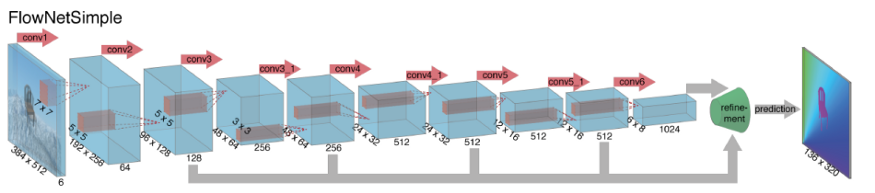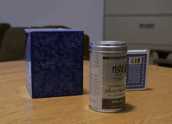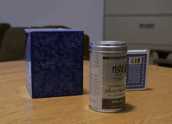# Robotics Perception Week 4

less than 1 minute read

## Nonlinear Least Squares [非线性最小二乘法]

• Method for non-linear least suqares problems - 2004
• Descent method
• The steepest descent method[最速下降]
• Newton’s method[牛顿下降]
• Linear Search[线性搜索]
• Trust Region and Damped Methods
• Non-linear least squares problems
• The Gauss-Newton Method
• The Levenberg-Marquardt Method
• Powell’s Dog leg Method
• A Hybrid Method: L-M and Quasi-Newton
• A Secant Version of the L-M Method
• A Secant Version of the Dog leg Method
• Final Remarks

## Optical Flow Method[光流法]

• 基本假设
• 亮度恒定不变， 由于需要对比两张连续的照片计算光流场，因此，如果亮度改变的话，图像匹配会存在误差
• 时间连续，或运动范围比较小，时间连续是为了让图像间的点满足对应关系
• 同一个点发生了位移，亮度应当是不变的
• 有$I(x,y,t)=I(x+dx, y=dy, t’)$
• 泰勒展开后得到 \begin{aligned} I(x,y,t)&=I(x+dx, y+dy, t+dt)\\ &=I(x,y,t) + I_xdx+I_ydy+I_tdt + R(x,y,t)&\text{R为高阶余项，当做0处理}\\ 0&=I_xdx+I_ydy+I_tdt \end{aligned}
• 其中
• $I_x, I_y, I_t$分别为光强对$x,y,t$的偏导数
• 偏导数的计算可以通过图像数据计算
• $dx, dy$为我们需要求的光流矢量，在一些教程中也会以$u,v$来表示，即$I_xu+I_yv+I_t=0$
• 为了求解$dx, dy$我们至少需要2个方程，即2个点，当点的数量超过2时，我们就需要用一些约束来求解最优解

### 光流法-不同的约束条件

#### 基于梯度的方法

• 利用图像的灰度的微分来计算速度矢量
• Horn-Schunck

#### 基于匹配的方法

• 特征匹配
• 根据目标特征点进行匹配
• 区域匹配
• 通过相似区域进行匹配

• 加个降采样

#### 基于相位的方法

• Fleet和Jepson，把光强数据改为相位

#### 神经动力学方法

• 利用神经网络建立视觉运动感知模型

#### 深度学习算法### 基于光流法的帧间插值

• 线性插值• 光流插值Categories:

Updated: